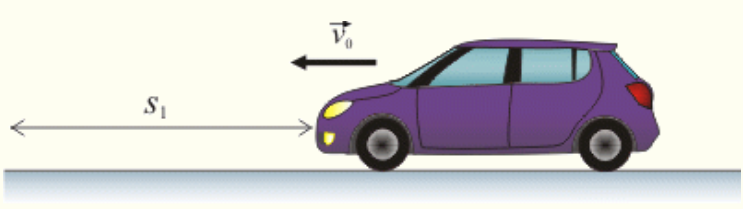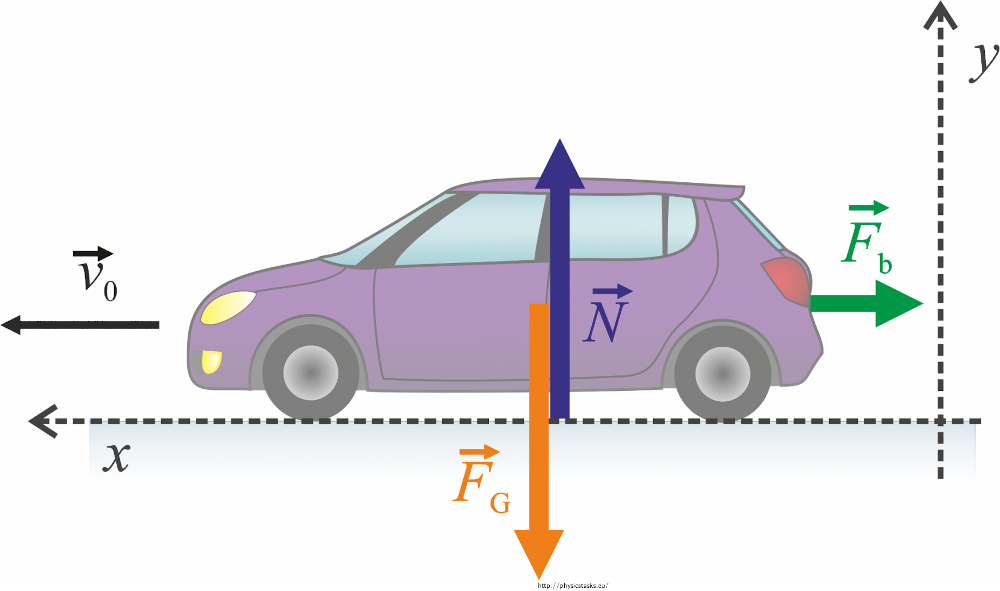When the brake pedal of a car moving 80 km·h-1 on a straight asphalt road is fully pressed, the car can stop in 50 m. How long will its braking distance be on an identical road which forms a 5° angle with the horizontal plane? Consider both the case when it brakes downhill and when it brakes uphill. Assume that at the time t = 0 s the coordinate is x = 0 m. The wheels do not slip. Neglect the air resistance.The equations from problem 30 are

 Enter Force breaking Enter mass Equation 3 result is Comment: Explanation in paragraphs and mathjaxs steps from 3 to 5 equation Enter initial speed Enter time equation 5 result is Comment: The explanation in paragraphs and mathjax steps from quation 5 to equation 7 Enter mass Enter time equation 7 result is Comment: The explanation in paragraphs and mathjax steps from quation 7 to equation 8 equation 8 result is Comment: